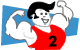# Patterns and ExponentsThe numbers 1, 2, 4, 8, 16, 32, 64, 128, 256, ... form a pattern. What is the rule for this pattern?   Answer

This list of numbers results from finding powers of 2 in sequence. Look at the table below and you will see several patterns.

 Exponential Form Factor Form Standard Form 20 = Any number (except 0) raised to the zero power is always equal to 1. 1 21 = Any number raised to the first power is always equal to itself. 2 22 = 2 x 2 = 4 23 = 2 x 2 x 2 = 8 24 = 2 x 2 x 2 x 2 = 16 25 = 2 x 2 x 2 x 2 x 2 = 32 26 = 2 x 2 x 2 x 2 x 2 x 2 = 64 27 = 2 x 2 x 2 x 2 x 2 x 2 x 2 = 128 28 = 2 x 2 x 2 x 2 x 2 x 2 x 2 x 2 = 256

Can you predict the next two numbers in the list after 256?   Answer

Example 1: Rewrite the numbers 1, 3, 9, 27, 81, 243, ... as a powers of 3.

Solution:

 Exponential Form Standard Form 30 = 1 31 = 3 32 = 9 33 = 27 34 = 81 35 = 243

Can you predict the next two numbers in the list after 243?   Answer

Example 2: If 73 = 343, then find 74 with only one multiplication.

Solution: 74 = 73 times 7

74 = 343 x 7

74 = 2,401

Example 3: If 45 = 1,024, then find 46 with only one multiplication.

Solution: 46 = 45 times 4

46 = 1,024 x 4

46 = 4,096

Example 4: If 100 = 1,   and 101 = 10,   and 102 = 100,   and 103 = 1,000,   then predict the values of 106 and 108 in standard form.

Solution:

 Exponential Form Standard Form Description 100 = 1 The exponent is 0; the number 1 has no zeros. 101 = 10 The exponent is 1; the number 10 has 1 zero. 102 = 100 The exponent is 2; the number 100 has 2 zeros. 103 = 1,000 The exponent is 3; the number 1,000 has 3 zeros. 106 = 1,000,000 The exponent is 6; the number 1,000,000 has 6 zeros. 108 = 100,000,000 The exponent is 8; the number 100,000,000 has 8 zeros.

Summary: When you find powers of a number in sequence, the resulting list of products forms a pattern. By examining this pattern, we can predict the next product in the list. Given the standard form of a number raised to the nth power, we can find the standard form of that number raised to the n+1 power with a single multiplication. When you find powers of 10 in sequence, a pattern of zeros is formed in the resulting list of products.

### Exercises

Directions: Read each question below. Click once in an ANSWER BOX and type in your answer; then click ENTER. Do not include commas in your answers. After you click ENTER, a message will appear in the RESULTS BOX to indicate whether your answer is correct or incorrect. To start over, click CLEAR.

 1 The numbers 1, 5, 25, 125, 625, ... are each powers of what number? ANSWER BOX:   RESULTS BOX:
 2 In Exercise 1, what is the next number in the list? ANSWER BOX:   RESULTS BOX:
 3 The numbers 1, 6, 36, 216, 1296, ... are each powers of what number? ANSWER BOX:   RESULTS BOX:
 4 10,000,000,000,000 is 10 raised to what power? ANSWER BOX:   RESULTS BOX:
 5 If 14 is equal to 1, then what is 1100? ANSWER BOX:   RESULTS BOX: